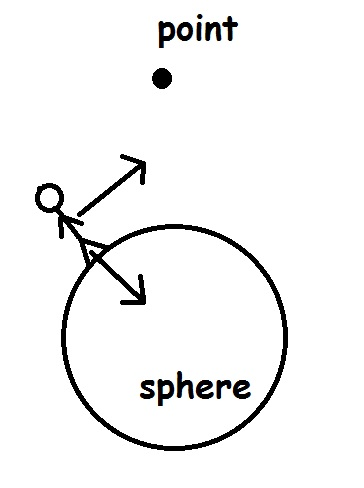# 2 axis rotated towards 2 different points

Hello everyone,

I’ve been cracking my head over this for a while now, so I thought why not ask the creative awesome people on the unity forums?

I have a situation where the player is walking around a spherical object, the y-axis should be the vector between the position of the player and the center of the sphere. Then he should rotate around the y-axis towards a point without messing up the rotation on the spherical object.

I’ve written the code for both seperately, but they can’t be used combined (at least not the way I’ve tried)

``````var direction;
var lookRotation:Quaternion;
var improved_rotation_position =    Vector3(hit.point.x,transform.transform.position.y,hit.point.z);
direction = (improved_rotation_position - transform.position).normalized;
lookRotation = Quaternion.LookRotation(direction);
``````

And the other code for the spherical object:

``````var bodyUp:Vector3 = transform.up;
var gravityUp:Vector3 = (transform.position-gravityTarget.transform.position).normalized;
var targetRotation:Quaternion = Quaternion.FromToRotation(bodyUp,gravityUp)*transform.rotation;
``````

Multiplying didn’t work (as I thought). Maybe I should work with eulerAngles only? Some help would be great thanks!

picture:note that the point is not on the Z- axis of the character but it should be looking towards it on that angle alone.

EDIT: Solution

Here is a easy way to get a lookat with one axis using eulerAngles:

To get the angle between 2 Vectors use

``````Vector3.Angle(V1,V2);
``````

note that it measures the angle between the 2 vectors with the middle point being Vector3(0,0,0).
In order to get it to use a V3 as middle point chance the upper code in this:

Vector3.Angle(V1-V3,V2-V3);

now we have the angle. There is a smaller problem that can arise using this, but with some clever thinking you can solve it. (I don’t want to spoil the ending)

result:

``````transform.eulerAngles = Vector3(transform.eulerAngles.x,transform.eulerAngles.y+angle,transform.eulerAngles.z); // for rotation around the axis
``````

In a situation like this where you need to rotate on only 1 axis, use Euler angles (360 degrees).

``````Vector3 MyEuler = transform.eulerAngles;
MyEuler.y += 10 * Time.deltaTime;
transform.eulerAngles = MyEuler;
``````

To rotate to a specific vector on the same plane, first you have to find the angle that’s needed. This can be done by subtracting the target point’s X and Y coordinates from your coordinate and getting the 2 sides of a triangle. You know that it’s a right angle triangle so you can just use the inverse tangent of the triangle’s sides to solve for the angle needed (feel free to ask for more explanation on this if it’s needed).

Use this to get the desired angle:

``````TargetRotation = Direction != Vector2d.zero ? Mathd.Atan (DirNorm.y / DirNorm.x) : TargetRotation;
``````

After you get the desired angle, you have to consider angle wrap around. Use a normalization method for your angles:

``````void NormalizeAngle(float Angle)
{
int Charge = Mathf.Abs(Angle) > 180 ? -1 : 1;
Angle = Charge * (Angle % 180);
}
``````

After you normalize your angles, simply lerp your current MyEuler.y to your desired angle or use some sort of velocity system.